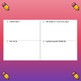# Expressions and Equations PracticeSubject
Resource Type
File Type

PDF

(370 KB|36 pages)
Product Rating
Standards
• Product Description
• StandardsNEW

Have students practice expressions and equations, while helping them practice single and multi-step word problems.

Word problems are difficult at times for students to understand, however, with regular practice students will become more successful.

This document is broken down into five days.

Day 1: Write Expressions. Included are 8 basic practice questions followed by 2 word problems.

Day 2: Evaluate Expressions. Included are 8 basic practice questions followed by 2 word problems.

Day 3: Write Equations. Included are 8 basic practice questions followed by 2 word problems.

Day 4: Solve multi-step word problems. Included are 6 word problems for students to complete.

Day 5: Quiz. Included are 6 basic problems and 1 word problem.

This document can be used to teach new skills to third grade students, as well as, providing review and practice for students in fourth grade who may need a refresher on expressions and equations.

Solve two-step word problems using the four operations. Represent these problems using equations with a letter standing for the unknown quantity. Assess the reasonableness of answers using mental computation and estimation strategies including rounding.
Understand division as an unknown-factor problem. For example, find 32 ÷ 8 by finding the number that makes 32 when multiplied by 8.
Determine the unknown whole number in a multiplication or division equation relating three whole numbers. For example, determine the unknown number that makes the equation true in each of the equations 8 × ? = 48, 5 = __ ÷ 3, 6 × 6 = ?.
Interpret whole-number quotients of whole numbers, e.g., interpret 56 ÷ 8 as the number of objects in each share when 56 objects are partitioned equally into 8 shares, or as a number of shares when 56 objects are partitioned into equal shares of 8 objects each. For example, describe a context in which a number of shares or a number of groups can be expressed as 56 ÷ 8.
Interpret products of whole numbers, e.g., interpret 5 × 7 as the total number of objects in 5 groups of 7 objects each. For example, describe a context in which a total number of objects can be expressed as 5 × 7.
Total Pages
36 pages
Included
Teaching Duration
N/A
Report this Resource to TpT
Reported resources will be reviewed by our team. Report this resource to let us know if this resource violates TpT’s content guidelines.